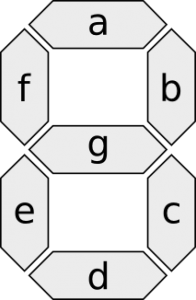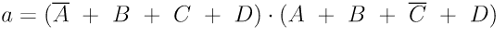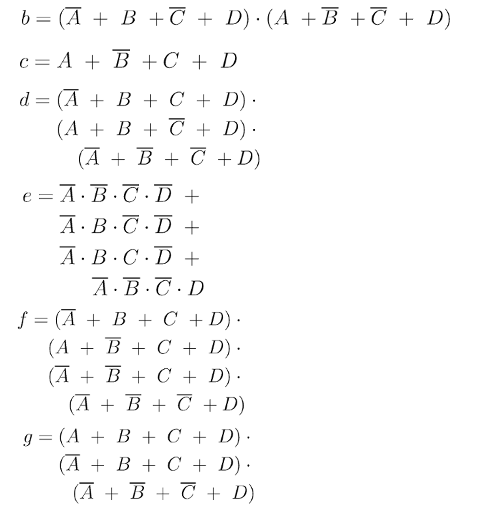This article is about creating a 7-segment display decoder in software. While this is probably considered a rather trivial task, it will serve as a good example for the discipline of Test-Driven Development (TDD). The motivation for a test-driven approach in this case is simple: being able to refactor later without the need to debug on hardware.

Let's start by first examining the seven-segment display. As the respective Wikipedia article says, it consists of seven LEDs that can be driven individually. Each LED pin (either anode or cathode, depending on the flavor) is assigned a letter a through g.[image based on the Wikipedia drawing by H2g2bob(CC BY-SA 3.0)]

All we need to get started is a truth table that states how the input digits 0 through 9 are mapped to the seven outputs. It is also possible to display (hex) digits a through f, but we will leave that out for the sake of simplicity. Instead of writing down the entire table, let's just put that into a unit test.

## TDD approach

Instead of implementing everything at once and test it later on, let's do it for each digit individually. After following that disciplice, we will end up with two things: a test case that resembles the truth table and the corresponding production code that makes that test pass (see the two listings below).

This is a pretty good example for a pattern that arises sometimes (to me at least). Because test code and production code have a 1:1 mapping, the production code isn't any more generic than the test code.

This feels weird to me and makes me think about how much sense a test-driven approach actually makes in such a scenario. Maybe testing after the fact would be sufficient here. What do you think?

## A (somewhat arbitrary) Refactoring

Anyways, to demonstrate the value of the test-driven approach chosen, let's do a refactoring of the production code. The idea is to construct a boolean expression for each of the output bits a through g. This is just another representation of the truth table from above and this approach is pretty common for creating logical circuits.

The set of inputs is represented as a 4-digit binary number consisting of bits A through D. Then a column for each output bit is added, as shown in the table below (note that all bits are sorted most-significant first).

Let's now create a boolean expression for output a. By looking at all rows from the table where output a is '0', we can establish an output equation in Full Conjunctive Form (CNF) (composed of "ANDs of ORs"):The CNF is chosen here because there are more '1's than '0's in the table. The same can be done for output b through g, utilizing either the CNF or DNF representation:Now here comes the interesting part. We put all the output equations right into the decode() function, replacing the previous implementation:

As the structure of the code has been changed while maintaining identical behavior, we expect the test to pass. And it does! I've prepared a repository containing the code at github.com/ronalterde/sevenseg.

## Conclusion

I'm not sure if the refactoring made is actually an improvement. Probably the initial version of the production code is more readable. But still, it shows that using the TDD approach we are able to make sure we don't break anything. Without debugging on hardware of course.

What do you think about the initial 1:1 mapping of the test and production code? That still feels awkward to me. Contact me on twitter: @ronalterde.